Checkout JEE MAINS 2022 Question Paper Analysis : Checkout JEE MAINS 2022 Question Paper Analysis :

# Graphic Representation Of Inverse Trigonometric Function

Graphic representation of inverse trigonometric functions: Inverse trigonometric functions are the functions that are also called arc functions. These are arcsine, arccosine, arctangent, arc-secant, arc-cotangent and arc-cosecant. Just like the trigonometric functions, we can also represent graphs of inverse trigonometric functions.

Basically, sin, cos and tan functions are used to find the length of the side of the right triangle, when we know the length of one side and measure of one of the acute angle. But, in the case of inverse trig functions, we basically find the measure of the angle, when the length of the two sides is known to us.

Also, see: Inverse Trigonometric Functions

Before we go ahead with the graphical representation, let us see the formulas for these functions.

## Inverse Trigonometric Function Formula

 Function Domain Range of an Inverse Function sin-1x(arcsinex) -1≤ x ≤1 -π/2≤y≤π/2 cos-1x(arcosinex) -1≤ x ≤1 0≤y ≤π tan-1x(arctangentx) – ∞ < x <  ∞ -π/2

Considering the domain and range of the inverse functions, following formulas are important to be noted:

• sin(sin-1x) = x, if -1 ≤ x ≤ 1 and sin-1(sin y) = y if -π/2 ≤ y ≤ π/2.
• cos(cos-1x) = x, if -1 ≤ x ≤ 1 and cos-1(cos y) = y if 0 ≤ y ≤ π.
• tan(tan-1x) = x, if -∞ < x < ∞ and cos-1(cos y) = y if -π/2 ≤ y ≤ π/2.
• cot(cot-1x) = x, if -∞ < x <  ∞ and cot-1(coy) = y if 0 < y <π.
• sec(sec-1x) = x, if -∞ ≤ x ≤ -1 or 1 ≤ x ≤ ∞ and sec-1(sey)= y if -0 ≤ y ≤ π, y ≠ π/2.
• cosec(cosec-1x) = x, if -∞ ≤ x ≤- 1 or 1 ≤ x ≤ ∞ and cosec-1(cosey) = y if -π/2 ≤ y ≤ π/2, y ≠ 0.

NOTE: Inverse trigonometric functions are also called “Arc Functions”, since, for a given value of a trigonometric function, they produce the length of arc needed to obtain that particular value.

## Graphs of Inverse Trigonometric Functions

The graphs of all the inverse trigonometric functions are given as follow.

### Graph of arcsine function

Arcsine function is inverse of the sine function denoted by sin-1 x. It is represented in the graph as shown below: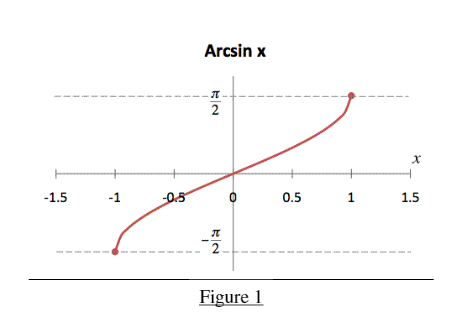### Graph of arccosine function

Arccosine function is inverse of the cosine function denoted by cos-1x. It is represented in the graph as shown below:### Graph of arctangent function

Arctangent function is inverse of the tangent function denoted by tan-1x. It is represented in the graph as shown below:### Graph of arccotangent function

Arccotangent function is inverse of the cotangent function denoted by cot-1x. It is represented in the graph as shown below: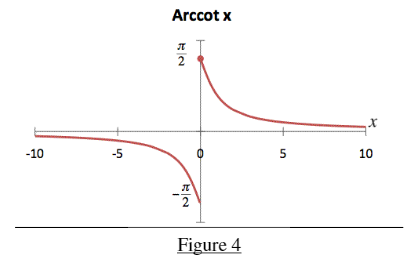### Graph of arcsecant function

Arcsecant function is the inverse of the secant function denoted by sec-1x. It is represented in the graph as shown below: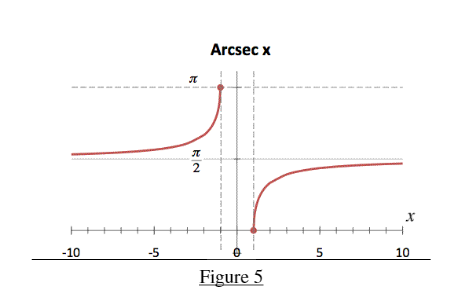### Graph of arccosecant function

Arccosecant function is inverse of the cosecant function denoted by cosec-1x. It is represented in the graph as shown below:## Video Lesson

### Graphic Representation Of Inverse Trigonometric FunctionAlso check:

### Inverse Trigonometric Functions### Multiple Angles## Circular representation of Inverse Trigonometric Functions

To solve most of the problems in Inverse Trigonometric Functions, it is very beneficial to understand the concept of circular representation of the trigonometric functions.

Let’s see an example of arcsinθ and arccosθ.

• Here the frame of reference is important. Moving forward, we would be assuming clockwise direction to be positive, and anti-clockwise direction to be negative.
With reference to the figure 7 and figure 8, point D is π/2, and E is -π/2. Point B is 0 and point C is π.
• Hence for figure 7, (sin-1θ) = θ and sin-1(-θ) = -sin-1(θ), taken in anticlockwise direction.Range ofarcsinθ is π/2 ≤ θ ≤ π/2and is taken from OE to OD in an anti-clockwise circular direction.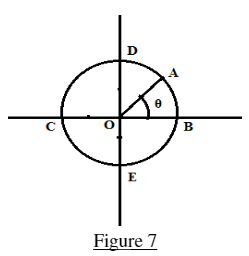Consider the inverse function cos-1(-θ) = π– θ

(Range of cosine function is 0 ≤ θ≤ π)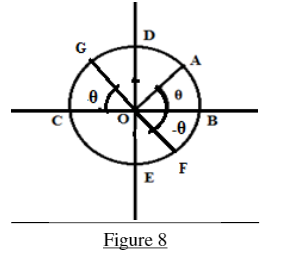(-)θ is taken as the clockwise direction which is represented as OF.

A range of cosine function is 0 ≤ θ ≤ π; from figure 8 –vertically opposite angles are equal that is Angle COG = Angle FOB.
The required distance travelled in the anti-clockwise direction to reach from OB to OG is π – θ. Hence, cos-1(-θ) = π – θ.

### Applications of Graph of Inverse Trigonometric Function

Consider, y = sin-1x + cos-1x

y = sin-1x + cos-1x; x ∈ [−1,1]

y = π/2

Therefore, graphical representation of y = sin-1x + cos-1is y =π/2 for x∈[−1,1] is given below: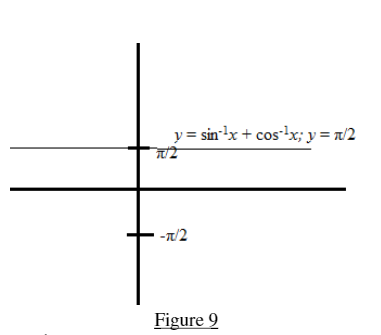Similarly, y = tan-1x + cot-1x

tan-1x + cot-1x = tan-1x + cot-1x = π/2

Therefore, graphical representation of y = tan-1x + cot-1is,With the help of inverse trigonometric functions represented graphically, we find that learning of topic becomes more easy, and more easy to explore.

The related subtopics will explain further details on the chapter of Inverse Trigonometric Functions & Trigonometry and will be as interesting and informative with BYJU’S.